# SilverZone - IOM PDF Sample Papers for Class 1

Class 1 sample paper & practice questions for International Olympiad of Mathematics (IOM) level 1 are given below. Syllabus for level 1 is also mentioned for these exams. You can refer these sample paper & quiz for preparing for the exam.#### Resources:

##### Sample Questions from Olympiad Success:
 Q.1 Q.2 Q.3 Q.4 Q.5 Q.6 Q.7 Q.8 Q.9 Q.10
 Q.1 What is the sum of all sides given in the figure?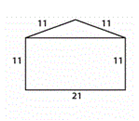a) 44 b) 55 c) 65 d) 75
 Q.2 How many books are there in the given figure?a) 12 b) 7 c) 10 d) 16
 Q.3 Which of the following number is missing in the image?a) 99 b) 15 c) 88 d) 51
 Q.4 How many pencils are there in the given figure?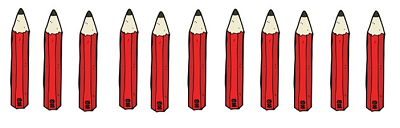a) 12 b) 7 c) 11 d) 16
 Q.5 Write the time for the given clock.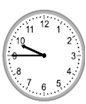a) 10:45 b) 9:45 c) 9:50 d) 10:40
 Q.6 Which of the following number matches the dots given here?a) 15 b) 16 c) 18 d) 20
 Q.7 Solve the equation: 20 + 19 - 59 + 30 = …………… a) 15 b) 10 c) 98 d) 12
 Q.8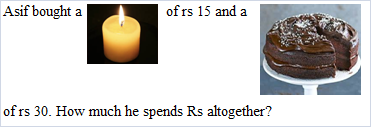a) Rs. 40 b) Rs. 35 c) Rs. 50 d) Rs. 45
 Q.9 Add the given numbers: 41 + 68 + 79 = …………….. a) 55 b) 57 c) 188 d) 108
 Q.10 Find the weight of each object?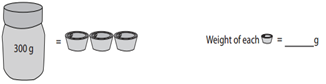a) 100 b) 125 c) 150 d) 300Sample PDF of SilverZone - International Olympiad of Mathematics (IOM) PDF Sample Papers for Class 1:

 Q.1 )c Q.2 )c Q.3 )a Q.4 )c Q.5 )b Q.6 )b Q.7 )b Q.8 )d Q.9 )c Q.10 )a

Q.1 : c | Q.2 : c | Q.3 : a | Q.4 : c | Q.5 : b | Q.6 : b | Q.7 : b | Q.8 : d | Q.9 : c | Q.10 : a